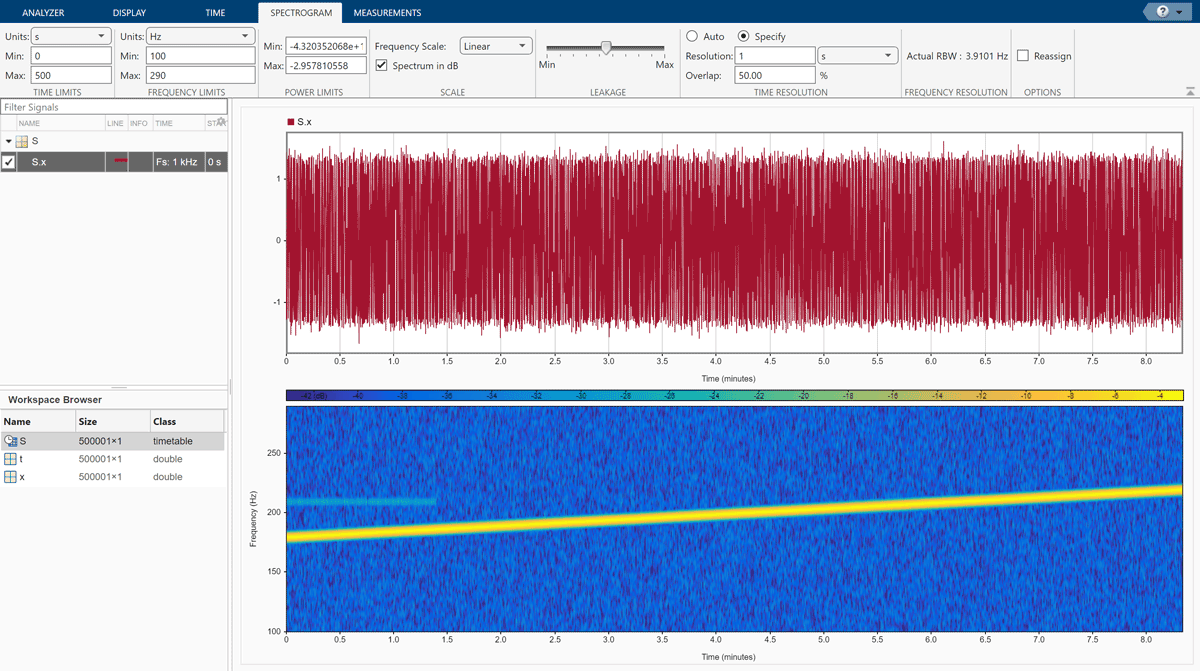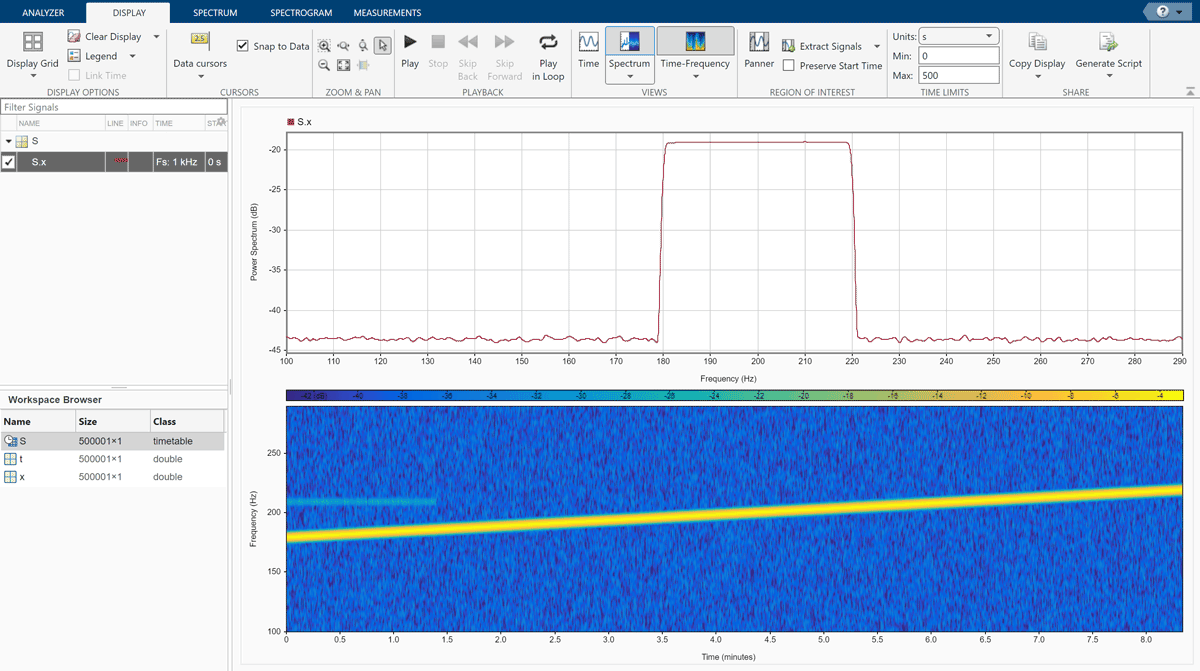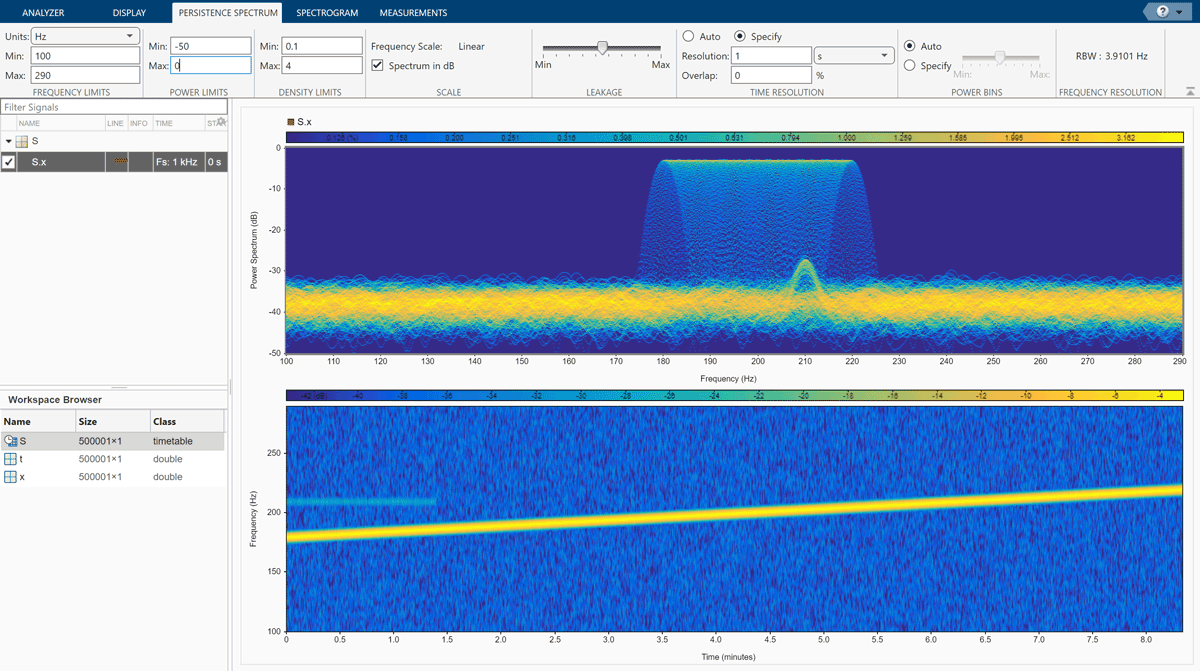# Find Interference Using Persistence Spectrum

Visualize an interference narrowband signal embedded in a broadband signal.

Generate a chirp sampled at 1 kHz for 500 seconds. The frequency of the chirp increases from 180 Hz to 220 Hz during the measurement.

```fs = 1000; t = (0:1/fs:500)'; x = chirp(t,180,t(end),220) + 0.15*randn(size(t));```

The signal also contains a 210 Hz sinusoid. The sinusoid has an amplitude of 0.05 and is present only for 1/6 of the total signal duration.

```idx = floor(length(x)/6); x(1:idx) = x(1:idx) + 0.05*cos(2*pi*t(1:idx)*210);```

Save the signal as a MATLAB® timetable.

`S = timetable(seconds(t),x);`

Open Signal Analyzer and drag the timetable from the Workspace Browser to a display. Click the Time-Frequency button to add a spectrogram view. On the Spectrogram tab, under Time Resolution, select Specify and enter a time resolution of 1 second. Set the Frequency Limits to 100 Hz and 290 Hz. Both signal components are visible.Go back to the Display tab. Click the Time button to remove the time view and click the Spectrum button to add a power spectrum view. The frequency range continues to be from 100 Hz to 290 Hz. The weak sinusoid is obscured by the chirp.Click the arrow under the Spectrum button to change the `Spectrum` view to a `Persistence Spectrum` view. On the Persistence Spectrum tab, under Time Resolution, select Specify and enter a time resolution of 1 second. Specify zero overlap between adjoining segments. Set the Power Limits to –50 dB and 0 dB and the Density Limits to 0.1 and 4. Now both signal components are clearly visible.On the Display tab, under Share, click Generate Script and select `Persistence Spectrum Script`. The script appears in the MATLAB Editor.

```% Compute persistence spectrum % Generated by MATLAB(R) 9.7 and Signal Processing Toolbox 8.2. % Generated on: 26-Dec-2018 16:07:45 % Parameters timeLimits = seconds([0 500]); % seconds frequencyLimits = [100 290]; % Hz timeResolution = 1; % seconds overlapPercent = 0; %% % Index into signal time region of interest S_x_ROI = S(:,'x'); S_x_ROI = S_x_ROI(timerange(timeLimits(1),timeLimits(2),'closed'),1); % Compute spectral estimate % Run the function call below without output arguments to plot the results [P,F,PWR] = pspectrum(S_x_ROI, ... 'persistence', ... 'FrequencyLimits',frequencyLimits, ... 'TimeResolution',timeResolution, ... 'OverlapPercent',overlapPercent);```